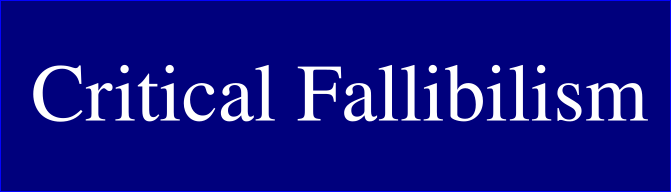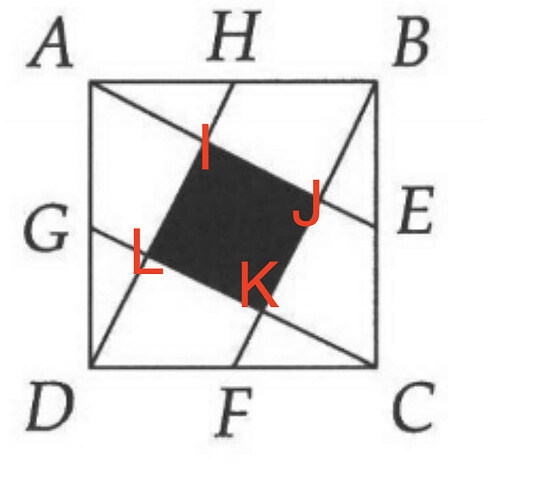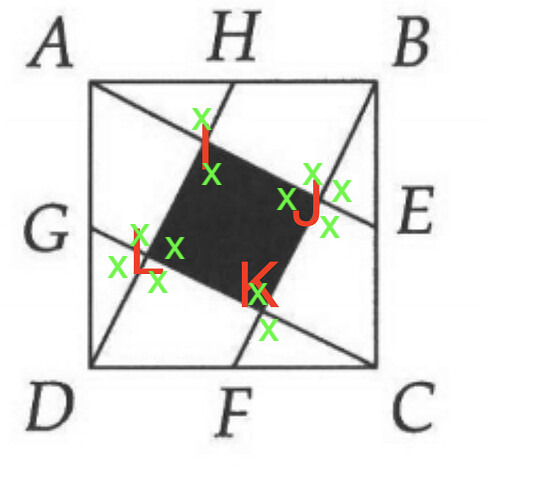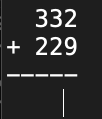# Justin Does Math

\overline{HD} starts from a corner - point D - and in diagonally going across a square with sides of length 20, arrives at a point (H) on the other side that is 10 to the right of where it started.

\overline{FB} starts at a midpoint - point F - and in diagonally going across the square, arrives at point B, which is to 10 to the right of where it started.

So the lines, in “traveling” across a square of length 20, both “shifted over” to the right 10. So they maintained an identical distance from each other throughout. This indicates that they’ll never meet, and are thus parallel.

Right. Another way to think of it is that they have the same slope.

(Btw, slope is another way that you can figure out that the angles in the middle are 90 degrees, but that would not have been covered in the book yet.)

1 Like\overline{AE} is a transversal crossing through parallel lines \overline{HD} and \overline{BF}. Therefore, the angles around point I have to match their corresponding angles around point J. For example, \angle JIL has to be the same as \angle EJK. The same logic applies for transversal \overline{GC} and points L and K.

\overline{HD} is a transversal through parallel lines \overline{AE} and \overline{GC}. Therefore, the angles around point I have to match their corresponding angles around point L. For example, \angle JIL has to be the same as \angle KLD. The same logic applies for transversal \overline{BF} and points J and K.

So consider internal angle \angle JIL. That angle has some value, x. The opposite angle to it, \angle AIH, has to be x. Also, because of the interlocking transversals, \angle KJE and \angle DLK and \angle FKC must be x. Because of opposite angles, \angle IJB and \angle GLI and \angle JKL must be x.

The congruence of the triangles means that \angle FKC must have the same value as \angle GLD and \angle EJB - namely, x. Opposite angles means that \angle ILK and \angle IJK must likewise be x. Thus the inside of the shaded area is 4x. We also see that around points L and J we have 4 x’s.The total of all the angles in full rotation around some point has to equal 360 degrees. Therefore, we know that 4x = 360, and we know that the interior of our shaded area has angles that equal 4x. x = 90, so the shaded area is a square.

That’s a good start. I think it’d be worth coming up with some more answers before moving on to some other problems like adding two larger numbers or subtracting or multiplying two small numbers.

V. Starting with one number as the difference between the combined number and the other number.
Step 1: 3 = 5 - 2
Step 2: 2 + 3 = 5

Step 1: 2 = 5 - 3
Step 2: 2 + 3 = 5

VI. Representation as individual squares

2 = \square \square
3 = \square \square \square
\square \square + \square \square \square = \square \square \square \square \square or 5

VII. Representation as Tally Marks

|| + ||| = ||||

Multiplying two small numbers:

I. 2 \times 6 = 12

II. With 6 broken down into prime factors:
2 \times 2 \times 3 = 12

First variation:
6 + 6 =
=6(1 + 1)
=6 \times 2

Second variation:
2 + 2 + 2 + 2 + 2 + 2
=2 (1 + 1 + 1 + 1 + 1 + 1)
= 2(6)

IV. As boxes with long rows

\square \square \square \square \square \square
\square \square \square \square \square \square

V. As boxes with tall columns

\square \square
\square \square
\square \square
\square \square
\square \square
\square \square

getting lots of mileage out of $\square$ herethought maybe going through some of the geometry stuff more carefully would be worth some effort

I’m trying a different way of doing things: do the entire chapter privately but publish “selections” consisting of only the problems and material that I think is particularly interesting for some reason (cuz the solution is interesting, cuz I got it wrong in an interesting way, whatever), and post that it publicly. I don’t want to have a bunch of private stuff on my blog. Also this involves me exercising a little more judgment about what might be interesting for other people to read and makes the posts themselves more readable due to being shorter. Crits on that idea welcome

332 + 229

I. A standard way they teach in school (or taught back in the day, at least, idk about now):Get 11 in the one’s column, carry a 1, get 6 in the ten’s column, 5 in the hundreds.
561.

II. Breaking up and rearranging:

(330 + 2) + (230 - 1)
rearrange: (330 + 230) + (2 - 1)
560 + 1
561

III. Variation on II

(300 + 30 + 2) + (200 + 20 + 9)
rearrange: (300 + 200) + (30 + 20) + (2 + 9)
500 + 50 + 11
561

229 is a prime number and has no common factors with 332 so there are some techniques we can’t use.

Tried to gather up some of the square problem ideas/solutions from this thread

I have been using this app a bit to draw geometry shapes. I like it

another post of selected exercises, this time from Chapter 10.3 of the Pre-Algebra AOPS book.

I think I’ll have a policy of sharing any problems I got wrong for any reason for now.

Since you have had trouble with the Geometry section in particular, I think it would benefit you to use their Introduction to Geometry book instead.

I looked at it a bit, and it has a lot of the same intro material & sections as the Prealgebra book you are using. It even has some of the same examples. But it explains things more than the Prealgebra book.

For example, Prealgebra starts its geometry section with 10.1, “Measuring Angles”. Introduction to Geometry has that same section in 2.2, but first it has 2.1, “What is an Angle?” And in Chapter 1 it has section 1.2, “Points, Lines, and Planes”, which is also relevant. I think I remember you said you weren’t sure what they meant by line segment, since they didn’t define it. In Introduction to Geometry, they actually do talk about that – they talk about drawing 2 points and say “If there were a straight path from one point to another, that path would be called a line segment, or just a segment.” (Hand-typed quote.)

1 Like

i will try it! thank you @ingracke!

This is a tangent, but I had initially written the second clause of this statement with:

I think it might actually benefit you to use their Introduction to Geometry book instead.

I removed a hedge: I changed “might actually” to “would”, since that is what I think. The statement still says “I think”, which I think is reasonable in this context. I am trying to give advice based on my own thoughts, not tell you what to do. And I don’t have full knowledge of your situation. (I’m less sure about when to use “I think” – all my statements are things I think, so that doesn’t have to be specified every single time. But there are times it is useful to specify.)

Regarding the topic, the reason I think it would be good is because you are trying to do beginner stuff and cover the topic thoroughly. So I assume you thought that the Prealgebra book would be a good place to start. But I think the Intro To Geometry actually covers the same intro material, but does it with more explanation and more beginner content.

1 Like

Yes.

That is good to know.